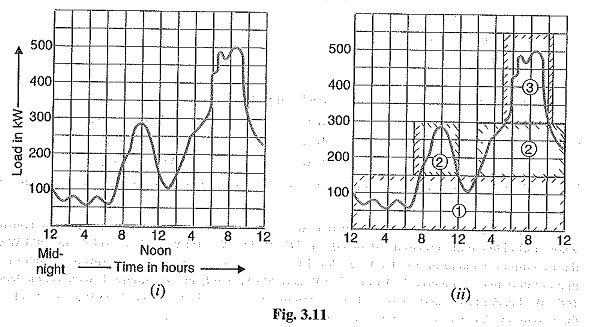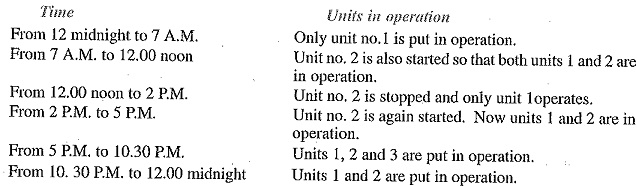## Selection of Power Generation Unit in Load Curve:

The load on a Power Generation Unit is seldom constant; it varies from time to time. Obviously, a single generating unit (i.e., alternator) will not be an economical proposition to meet this varying load. It is because a single unit will have very poor efficiency during the periods of light loads on the power station. Therefore, in actual practice, a number of generating units of different sizes are installed in a power station. The selection of the number and sizes of the units is decided from the annual load curve of the station. The number and size of the units are selected in such a way that they correctly fit the station load curve. Once this underlying principle is adhered to, it becomes possible to operate the generating units at or near the point of maximum efficiency.

The principle of selection of number and sizes of Power Generation Unit with the help of load curve is illustrated in Fig. 3.11. In Fig. 3.11 (i), the annual load curve of the station is shown. It is clear form the curve that load on the station has wide variations ; the minimum load being somewhat near 50 kW and maximum load reaching the value of 500 kW. It hardly needs any mention that use of a single unit to meet this varying load will be highly uneconomical.As discussed earlier, the total plant capacity is divided into several generating units of different sizes to fit the load curve. This is illustrated in Fig. 3.1100 where the plant capacity is divided into three units numbered as 1, 2 and 3. The cyan colour outline shows the units capacity being used. The three units employed have different capacities and are used according to the demand on the station. In this case, the operating schedule can be as under :Thus by selecting the proper number and sizes of units, the generating units can be made to operate near maximum efficiency. This results in the overall reduction in the cost of production of electrical energy.

### Important Points in the Selection of Units

While making the selection of number and sizes of the generating units, the following points should be kept in view :

• The number and sizes of the units should be so selected that they approximately fit the annual load curve of the station.
• The units should be preferably of different capacities to meet the load requirements. Although use of identical units (i.e., having same capacity) ensures saving in cost, they often do not meet the load requirement.
• The capacity of the plant should be made 15% to 20% more than the maximum demand to meet the future load requirements.
• There should be a spare generating unit so that repairs and overhauling of the working units can be carried out.
• The tendency to select a large number of units of smaller capacity in order to fit the load curve very accurately should be avoided. It is because the investment cost per kW of capacity increases as the size of the units decreases.
Scroll to Top